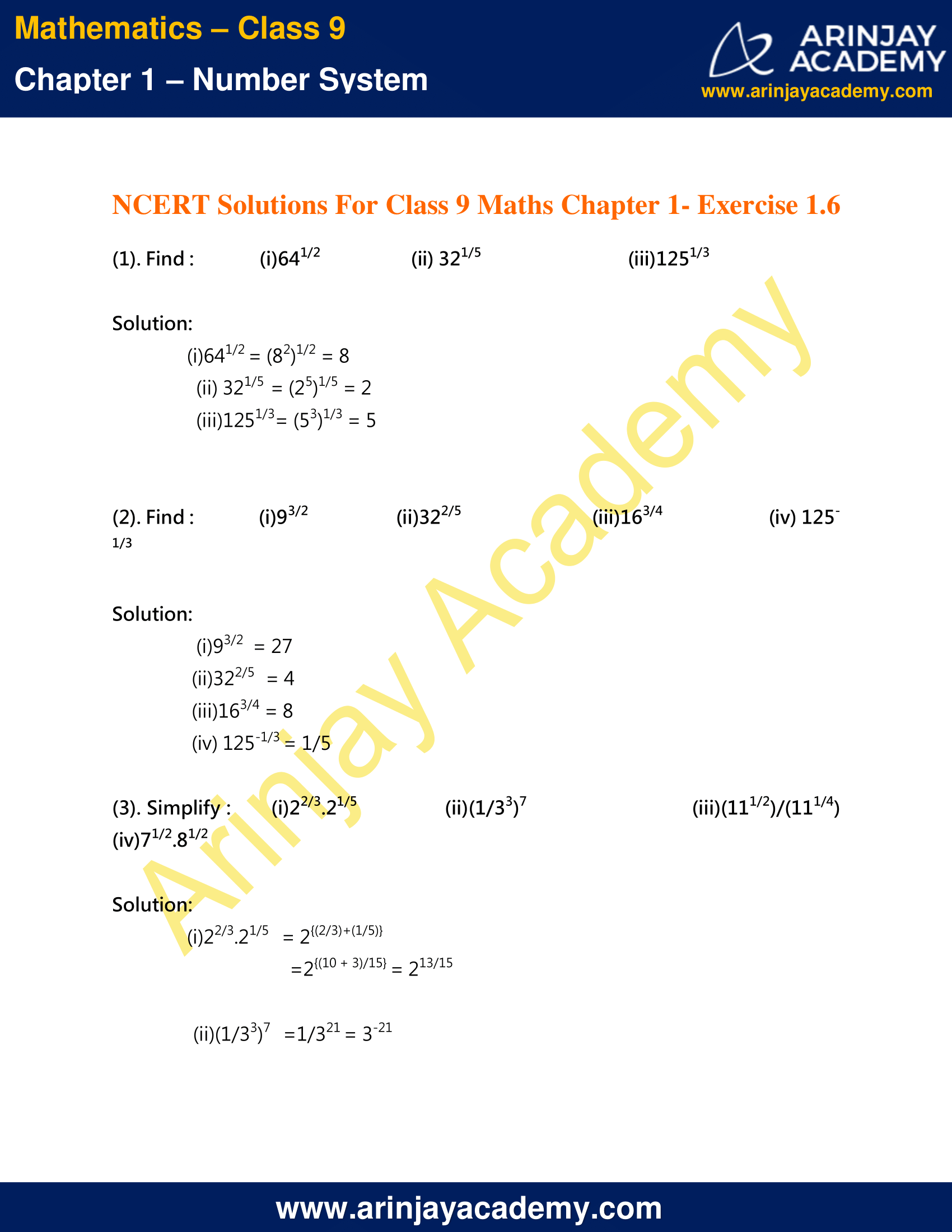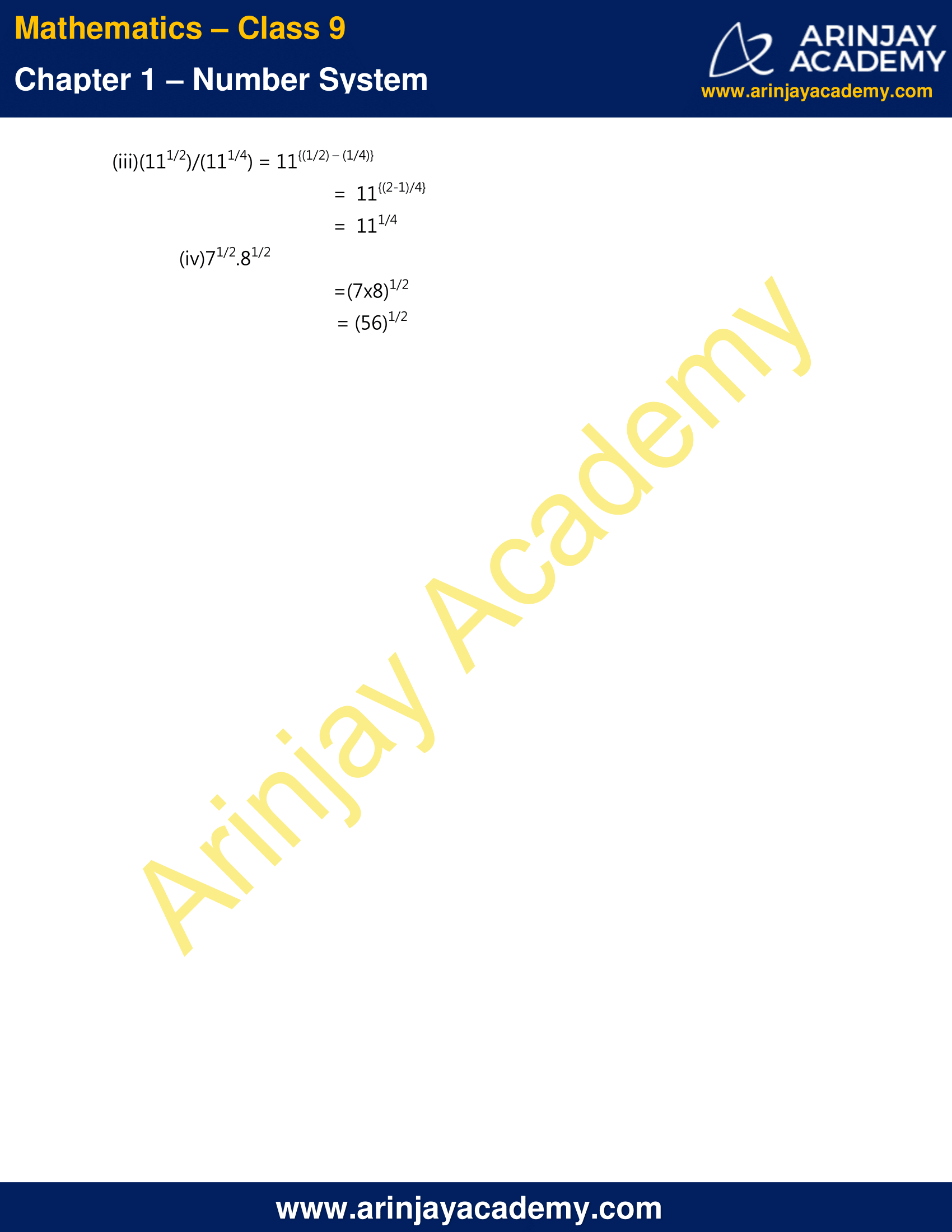# NCERT Solutions For Class 9 Maths Chapter 1 Exercise 1.6 – Number System

Download NCERT Solutions For Class 9 Maths Chapter 1 Exercise 1.6 – Number System. This Exercise contains 3 questions, for which detailed answers have been provided in this note. In case you are looking at studying the remaining Exercise for Class 9 for Maths NCERT solutions for Chapter 2 or other Chapters, you can click the link at the end of this Note.

### NCERT Solutions For Class 9 Maths Chapter 1 Exercise 1.6 – Number SystemNCERT Solutions For Class 9 Maths Chapter 1 Exercise 1.6 – Number System

(1). Find :

(i)641/2
(ii) 321/5
(iii)1251/3

Solution:

(i)641/2 = (82)1/2 = 8

(ii) 321/5  = (25)1/5 = 2

(iii)1251/3= (53)1/3 = 5

(2). Find :

(i)93/2
(ii)322/5
(iii)163/4
(iv) 125-1/3

Solution:

(i)93/2  = 27

(ii)322/5   = 4

(iii)163/4 = 8

(iv) 125-1/3 = 1/5

(3). Simplify :

(i)22/3.21/5
(ii)(1/33)7
(iii)(111/2)/(111/4)
(iv)71/2.81/2

Solution:

(i)22/3.21/5

= 2{(2/3)+(1/5)}

=2{(10 + 3)/15}

= 213/15

(ii)(1/33)7

=1/321

= 3-21

(iii)(111/2)/(111/4)

= 11{(1/2) – (1/4)}

=  11{(2-1)/4}

=  111/4

(iv)71/2.81/2

=(7×8)1/2

= (56)1/2

NCERT Solutions for Class 9 Maths Chapter 1 Exercise 1.6 – Number System, has been designed by the NCERT to test the knowledge of the student on the topic – Laws of Exponents for Real Numbers

The next Exercise for NCERT Solutions for Class 9 Maths Chapter 2 Exercise 2.1 – Number System can be accessed by clicking here.

Download NCERT Solutions For Class 9 Maths Chapter 1 Exercise 1.6 – Number System